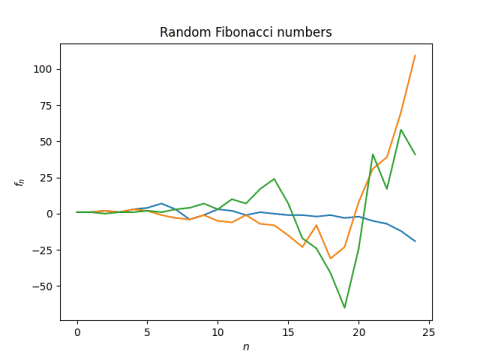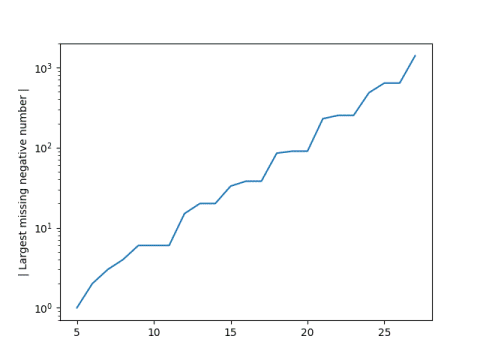# Is every number a random Fibonacci number?

The previous post looked at random Fibonacci sequences. These are defined by

f1 = f2 = 1,

and

fn = fn-1 ± fn-2

for n > 2, where the sign is chosen randomly to be +1 or -1.

Conjecture: Every integer can appear in a random Fibonacci sequence.

Here’s why I believe this might be true. The values in a random Fibonacci sequence of length n are bound between –Fn-3 and Fn. This range grows like On) where φ is the golden ratio. But the number of ways to pick + and – signs in a random Fibonacci equals 2n.

By the pigeon hole principle, some choices of signs must lead to the same numbers: if you put 2n balls in φn boxes, some boxes get more than one ball since φ < 2. That’s not quite rigorous since the range is  On) rather than exactly φn, but that’s the idea. The graph included in the previous post shows multiple examples where different random Fibonacci sequences overlap.Now the pigeon hole principle doesn’t show that the conjecture is true, but it suggests that there could be enough different sequences that it might be true. The fact that the ratio of balls to boxes grows exponentially doesn’t hurt either.

Empirically, it appears that as you look at longer and longer random Fibonacci sequences, gaps in the range are filled in.

The following graphs consider all random Fibonacci sequences of length n, plotting the smallest positive integer and the largest negative integer not in the range. For the negative integers, we take the absolute value. Both plots are on a log scale.

First positive number missing:Absolute value of first negative number missing:The span between the largest and smallest possible random Fibonacci sequence value is growing exponentially with n, and the range of consecutive numbers in the range is apparently also growing exponentially with n.

The following Python code was used to explore the gaps.

```    import numpy as np
from itertools import product

def random_fib_range(N):
r = set()
x = np.ones(N, dtype=int)
for signs in product((-1,1), repeat=(N-2)):
for i in range(2, N):
b = signs[i-2]
x[i] = x[i-1] + b*x[i-2]
return sorted(list(r))

def stats(r):
zero_location = r.index(0)

# r is sorted, so these are the min and max values
neg_gap = r  // minimum
pos_gap = r[-1] // maximum

for i in range(zero_location-1, -1, -1):
if r[i] != r[i+1] - 1:
neg_gap = r[i+1] - 1
break

for i in range(zero_location+1, len(r)):
if r[i] != r[i-1] + 1:
pos_gap = r[i-1] + 1
break

return  (neg_gap, pos_gap)

for N in range(5,25):
r = random_fib_range(N)
print(N, stats(r))
```

## Proof

Update: Nathan Hannon gives a simple proof of the conjecture by induction in the comments.

You can create the series (1, 2) and (1, 3). Now assume you can create (1, n). Then you can create (1, n+2) via (1, n, n+1, 1, n+2). So you can create any positive even number starting from (1, 2) and any odd positive number from (1, 3).

You can do something analogous for negative numbers via (1, n, n-1, -1, n-2, n-3, -1, 2-n, 3-n, 1, n-2).

This proof can be used to create an upper bound on the time required to hit a given integer, and a lower bound on the probability of hitting a given integer during a random Fibonacci sequence.

Nathan’s construction requires more steps to produce new negative numbers, but that is consistent with the range of random Fibonacci sequences being wider on the positive side, [-Fn-3, Fn].

***

 To minimize the random Fibonacci sequence, you can chose the signs so that the values are 1, 1, 0, -1, -1, -2, -3, -5, … Note that absolute value of this sequence is the ordinary Fibonacci sequence with 3 extra terms spliced in. That’s why the lower bound is –Fn-3.

## 3 thoughts on “Is every number a random Fibonacci number?”

1. Nathan Hannon

If you can form any sequence including (1, n), then you can form (1, n, n+1, -1, n+2, n+1, 1, n+2) or (1, n, n+1, -1, n, n-1, 1, n-2). Since you can obviously form (1, 1) and (1, 2), you can get any integer.

A stronger conjecture might be “The shortest time for an integer n to appear in a random Fibonacci sequence is O(log n).”

2. redxaxder

@Nathan:

You cannot form 1,n,n+1,-1 using this rule.

-1 is n – (n+1), but this rule only allows that position to equal (n+1) ± n.

3. Nathan Hannon

Sorry, I misread the rule. You can still do the same thing with

(1, n, n+1, 1, n+2)
(1, n, n-1, -1, n-2, n-3, -1, 2-n, 3-n, 1, n-2)